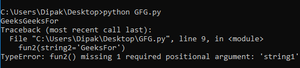Open in App
Not now

# How to pass optional parameters to a function in Python?

• Last Updated : 04 Sep, 2021

In Python, when we define functions with default values for certain parameters, it is said to have its arguments set as an option for the user. Users can either pass their values or can pretend the function to use theirs default values which are specified.

In this way, the user can call the function by either passing those optional parameters or just passing the required parameters.

There are two main ways to pass optional parameters in python

• Without using keyword arguments.
• By using keyword arguments.

## Passing without using keyword arguments

Some main point to be taken care while passing without using keyword arguments is :

• The order of parameters should be maintained i.e. the order in which parameters are defined in function should be maintained while calling the function.
• The values for the non-optional parameters should be passed otherwise it will throw an error.
• The value of the default arguments can be either passed or ignored.

Below are some codes which explain this concept.

Example 1:

## Python3

 `# Here b is predefined and hence is optional.` `def` `func(a, b``=``1098``):` `    ``return` `a``+``b`     `print``(func(``2``, ``2``))`   `# this 1 is represented as 'a' in the function and` `# function uses the default value of b` `print``(func(``1``))`

Output:

```4
1099```

Example 2: we can also pass strings.

## Python3

 `# Here string2 is the default string used` `def` `fun2(string1, string2``=``"Geeks"``):` `    ``print``(string1 ``+` `string2)`     `# calling the function using default value` `fun2(``'GeeksFor'``)`   `# calling without default value.` `fun2(``'GeeksFor'``, ``"Geeks"``)`

Output:

```GeeksForGeeks
GeeksForGeeks```

## Passing with keyword arguments

When functions are defined then the parameters are written in the form “datatype keyword-name”. So python provides a mechanism to call the function using the keyword name for passing the values. This helps the programmer by relieving them not to learn the sequence or the order in which the parameters are to be passed.

Some important points we need to remember are as follows:

• In this case, we are not required to maintain the order of passing the values.
• There should be no difference between the passed and declared keyword names.

Below is the code for its implementation.

## Python3

 `# Here string2 is the default string used` `def` `fun2(string1, string2``=``"Geeks"``):` `    ``print``(string1 ``+` `string2)`     `# Thiscan be a way where no order is needed.` `fun2(string2``=``'GeeksFor'``, string1``=``"Geeks"``)`   `# since we are not mentioning the non-default argument` `# so it will give error.` `fun2(string2``=``'GeeksFor'``)`

Output:As we can see that we don’t require any order to be maintained in the above example. Also, we can see that when we try to pass only the optional parameters then it raises an error. This happens since optional parameters can be omitted as they have a default with them, but we cannot omit required parameters (string1 in the above case.) Hence, it shows an error with the flag: “missing 1 required argument”.

This example will give a more insight idea of above topic:

## Python3

 `def` `func(a, b, c``=``'geeks'``):` `    ``print``(a, ``"type is"``, ``type``(a))` `    ``print``(b, ``"type is"``, ``type``(b))` `    ``print``(c, ``"type is"``, ``type``(c))`     `# The optional parameters will not decide` `# the type of parameter passed.` `# also the order is maintained` `print``(``"first call"``)` `func(``2``, ``'z'``, ``2.0``)`   `# below call uses the default` `# mentioned value of c` `print``(``"second call"``)` `func(``2``, ``1``)`   `# The below call (in comments) will give an error` `# since other required parameter is not passed.` `# func('a')` `print``(``"third call"``)` `func(c``=``2``, b``=``3``, a``=``'geeks'``)`

Output:

```first call
2 type is <class 'int'>
z type is <class 'str'>
2.0 type is <class 'float'>
second call
2 type is <class 'int'>
1 type is <class 'int'>
geeks type is <class 'str'>
third call
geeks type is <class 'str'>
3 type is <class 'int'>
2 type is <class 'int'>```

So basically python functional calls checks only if the required number of functional parameters are passed or not.

Below shows the case where a user tries to pass arguments in both ways discussed above along with the precaution given:

## Python3

 `def` `comp(a, b``=``2``):` `    ``if``(a < b):` `        ``print``(``"first parameter is smaller"``)` `    ``if``(a > b):` `        ``print``(``"second parameter is smaller"``)` `    ``if``(a ``=``=` `b):` `        ``print``(``"both are of equal value."``)`     `print``(``"first call"``)` `comp(``1``)` `print``(``"second call"``)` `comp(``2``, ``1``)` `print``(``"third call"``)` `comp(b``=``1``, a``=``-``1``)` `print``(``"fourth call"``)` `comp(``-``1``, b``=``0``)`

Output:

```first call
first parameter is smaller
second call
second parameter is smaller
third call
first parameter is smaller
fourth call
first parameter is smaller```

So one thing we should remember that the keyword argument should used after all positional arguments are passed. Hence this is an important thing we must keep in mind while passing parameters in both ways to same function.

My Personal Notes arrow_drop_up
Related Articles